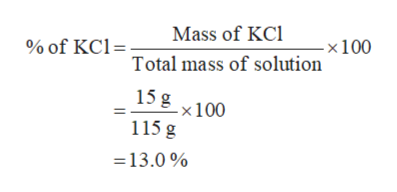# What is the mass percent of potassium chloride in a solution that is made by dissolving 15.0 g KCl in 100.0 g H2O? Show work

Question
1 views
What is the mass percent of potassium chloride in a solution that is made by dissolving 15.0 g KCl in 100.0 g H2O? Show work
check_circle

Step 1

Given:

Mass of water is 100 g.

Mass of KCl is 15.0 g.

Step 2

It is given that solution is made by dissolving 15 g of KCl in 100 g of water. Thus, total mass of a solution is,

=Mass of water + Mass of KCl

=100 g + 15 g

=115 g

Step 3

The percent of KCl in a solution is...help_outlineImage TranscriptioncloseMass of KCl % of KCl=: -×100 Total mass of solution 15 g -x100 115 g =13.0 % fullscreen

### Want to see the full answer?

See Solution

#### Want to see this answer and more?

Solutions are written by subject experts who are available 24/7. Questions are typically answered within 1 hour.*

See Solution
*Response times may vary by subject and question.
Tagged in

### Solutions